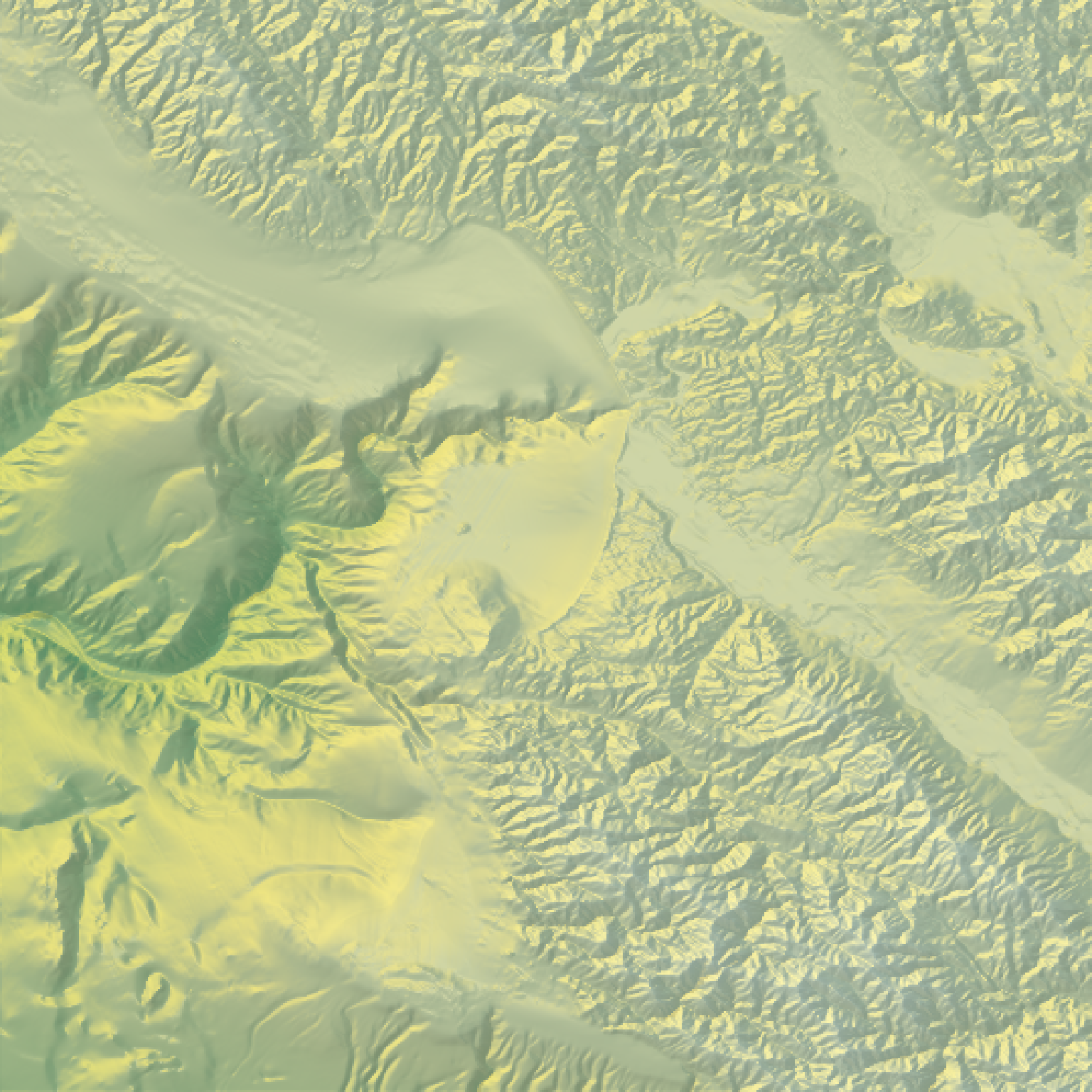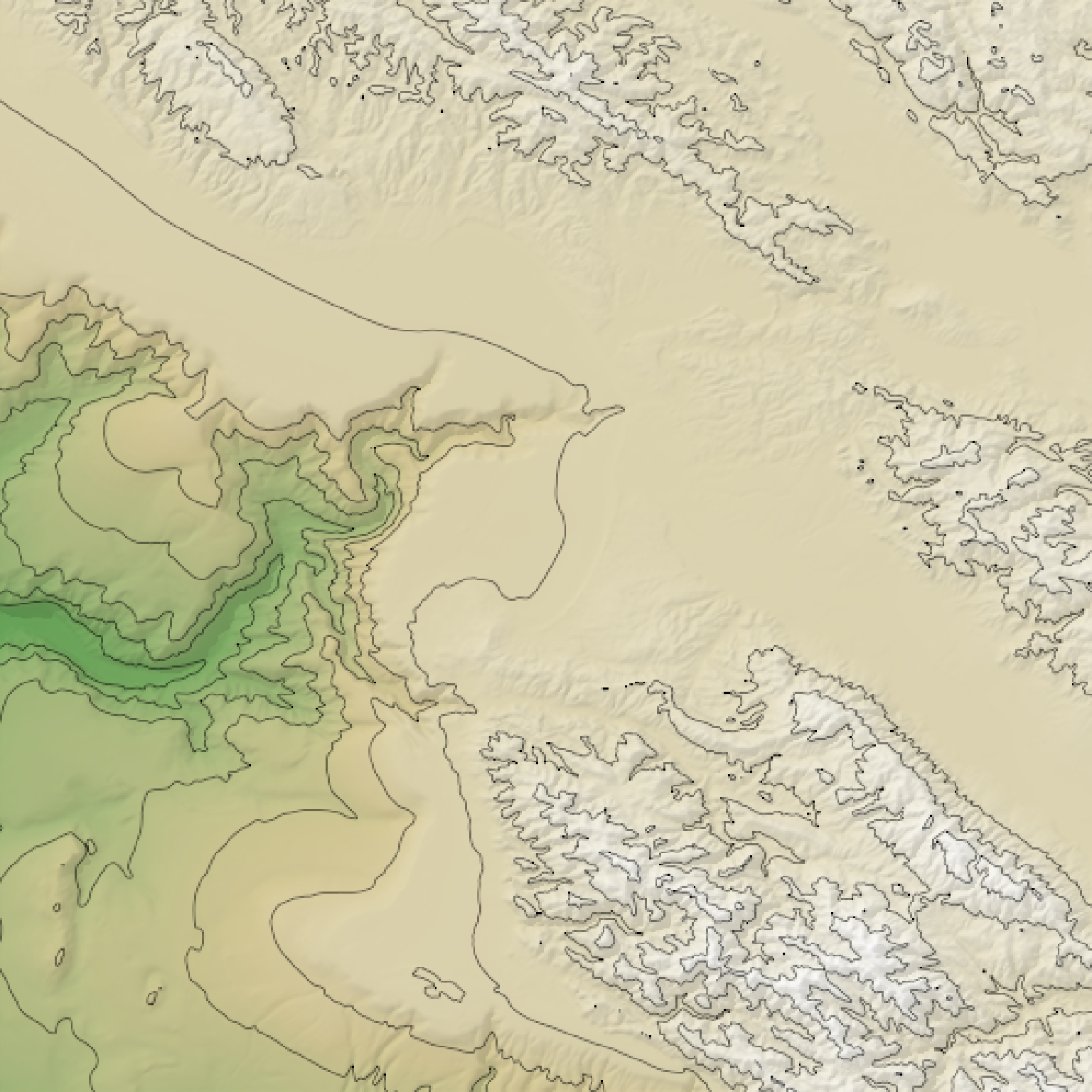Overlays an image (with a transparency layer) on the current map.

add_overlay(hillshade, overlay, alphacolor = NULL, alphalayer = 1,
alphamethod = "max", gamma_correction = TRUE)

## Arguments

hillshade A three-dimensional RGB array or 2D matrix of shadow intensities. A three or four dimensional RGB array, where the 4th dimension represents the alpha (transparency) channel. If the array is 3D, alphacolor should also be passed to indicate transparent regions. Default NULL. If overlay is a 3-layer array, this argument tells which color is interpretted as completely transparent. Default 1. Defines minimum tranparaency of layer. If transparency already exists in overlay, the way add_overlay combines the two is determined in argument alphamethod. Default max. Method for dealing with pre-existing transparency with layeralpha. If max, converts all alpha levels higher than layeralpha to the value set in layeralpha. If multiply, multiples all pre-existing alpha values with layeralpha. If none, keeps existing tranparencies and only changes opaque entries. Default TRUE. Controls gamma correction when adding colors. Default exponent of 2.2.

## Examples

#Here, we overlay a base R elevation plot with our raytraced shadow layer:

fliplr = function(x) {
x[,ncol(x):1]
}tempfilename = tempfile()
on.exit(par(old.par))
png(tempfilename,width = 401,height=401)
par(mar = c(0,0,0,0))
raster::image(fliplr(montereybay),axes = FALSE,col = rev(terrain.colors(1000)))
dev.off()#> pdf
plot_map()plot_map()